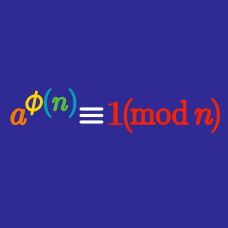Number Theory

# RSA

Solving which of the following problems would allow one to break RSA encryption?

Alice chooses a public key of $$n=187$$ and $$e=3$$. What is her private key?

Bob's public key is $$426759435605268851$$ and $$e=3$$. Alice uses ASCII encryption and sends the ciphertext $$c=298049520771754739$$. Which of the following was Alice's original message?

Mallory discovers many public keys, including the following:

• $$n=70441807$$, $$e=3$$
• $$n=10645627$$, $$e=17$$
• $$n=63339281$$, $$e=65537$$
• $$n=24864431$$, $$e=257$$
• $$n=89221291$$, $$e=17$$

What number can Mallory discover is the prime factor of one (or more) of these keys, without needing to factor any of them, making use of a vulnerability of RSA?

Knowing which of the following would allow an attacker to efficiently break the RSA encryption?

×

Problem Loading...

Note Loading...

Set Loading...